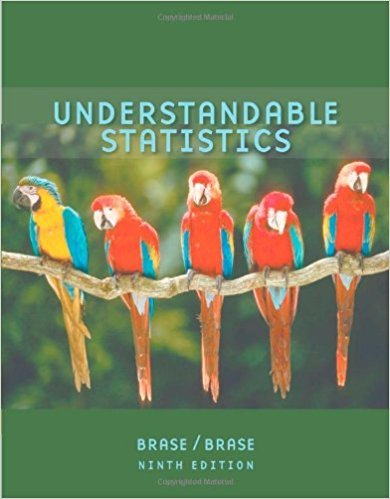×
×

# Statistical Literacy Suppose your data consist of aISBN: 9780618949922 213

## Solution for problem 2 Chapter 12.4

Understandable Statistics | 9th Edition

• Textbook Solutions
• 2901 Step-by-step solutions solved by professors and subject experts
• Get 24/7 help from StudySoup virtual teaching assistantsUnderstandable Statistics | 9th Edition

4 5 1 380 Reviews
29
5
Problem 2

Statistical Literacy Suppose your data consist of a sequence of numbers. To apply a runs test for randomness about the medium, what process do you use to convert the numbers into two distinct symbols?

Step-by-Step Solution:
Step 1 of 3

Applied Statistical Methods Chapter 2 Definitions/Notes Associations between Variables:  Two variables measured on the same cases are associated if knowing the value of one of the variables tells you something that you would not otherwise know about the value of the other variable  Response variable/dependent variable: variable being observed (or...

Step 2 of 3

Step 3 of 3

##### ISBN: 9780618949922

Unlock Textbook Solution